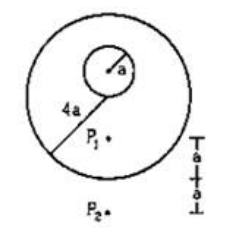# Solve the following :Question:

A uniform metal sphere of radius a and mass $\mathrm{M}$ is surrounded by a thin uniform spherical shell of equal mass and radius $4 a$ (figure 11-E2). The center of the shell falls on the surface of the inner sphere. Find the gravitational field at the points $P_{1}$ and $P_{2}$ shown in the figure.Solution:

(a) At point $P_{1}$

Net gravitational field=field due to shell+ field due to sphere

$\vec{E}_{N}=0+\frac{G M}{(a+4 a-a)^{2}}$

$\vec{E}_{N}=\frac{G M}{16 a^{2}}$

(b) At point $P_{2}$

Net gravitational field=field due to shell+ field due to sphere

$\vec{E}_{N}=\frac{G M}{(4 a+a)^{2}}+\frac{G M}{(4 a+a+a)^{2}}$

$\vec{E}_{N}=\frac{G M}{25 a^{2}}+\frac{G M}{36 a^{2}}$

$\vec{E}_{N}=\frac{61 G M}{900 a^{2}}$